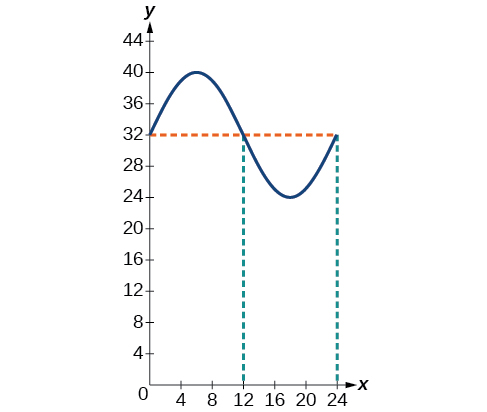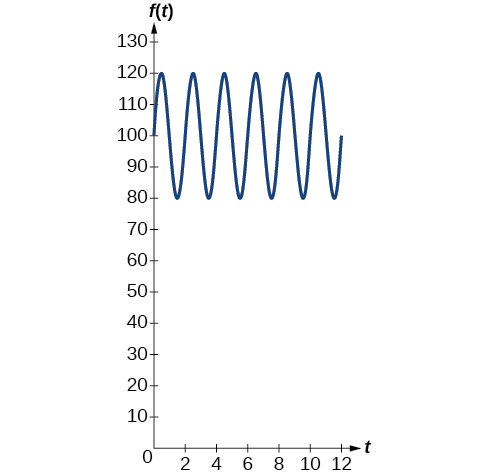# 7.6 Modeling with trigonometric equations  (Page 3/14)

 Page 3 / 14

## Describing periodic motion

The hour hand of the large clock on the wall in Union Station measures 24 inches in length. At noon, the tip of the hour hand is 30 inches from the ceiling. At 3 PM, the tip is 54 inches from the ceiling, and at 6 PM, 78 inches. At 9 PM, it is again 54 inches from the ceiling, and at midnight, the tip of the hour hand returns to its original position 30 inches from the ceiling. Let $\text{\hspace{0.17em}}y\text{\hspace{0.17em}}$ equal the distance from the tip of the hour hand to the ceiling $\text{\hspace{0.17em}}x\text{\hspace{0.17em}}$ hours after noon. Find the equation that models the motion of the clock and sketch the graph.

Begin by making a table of values as shown in [link] .

$x$ $y$ Points to plot
Noon 30 in $\left(0,30\right)$
3 PM 54 in $\left(3,54\right)$
6 PM 78 in $\left(6,78\right)$
9 PM 54 in $\left(9,54\right)$
Midnight 30 in $\left(12,30\right)$

To model an equation, we first need to find the amplitude.

The clock’s cycle repeats every 12 hours. Thus,

The vertical shift is

There is no horizontal shift, so $\text{\hspace{0.17em}}C=0.\text{\hspace{0.17em}}$ Since the function begins with the minimum value of $\text{\hspace{0.17em}}y\text{\hspace{0.17em}}$ when $\text{\hspace{0.17em}}x=0\text{\hspace{0.17em}}$ (as opposed to the maximum value), we will use the cosine function with the negative value for $\text{\hspace{0.17em}}A.\text{\hspace{0.17em}}$ In the form $\text{\hspace{0.17em}}y=A\text{\hspace{0.17em}}\mathrm{cos}\left(Bx±C\right)+D,\text{\hspace{0.17em}}$ the equation is

$y=-24\text{\hspace{0.17em}}\mathrm{cos}\left(\frac{\pi }{6}x\right)+54$

See [link] .

## Determining a model for tides

The height of the tide in a small beach town is measured along a seawall. Water levels oscillate between 7 feet at low tide and 15 feet at high tide. On a particular day, low tide occurred at 6 AM and high tide occurred at noon. Approximately every 12 hours, the cycle repeats. Find an equation to model the water levels.

As the water level varies from 7 ft to 15 ft, we can calculate the amplitude as

The cycle repeats every 12 hours; therefore, $\text{\hspace{0.17em}}B\text{\hspace{0.17em}}$ is

$\frac{2\pi }{12}=\frac{\pi }{6}$

There is a vertical translation of $\text{\hspace{0.17em}}\frac{\left(15+8\right)}{2}=11.5.\text{\hspace{0.17em}}$ Since the value of the function is at a maximum at $\text{\hspace{0.17em}}t=0,$ we will use the cosine function, with the positive value for $\text{\hspace{0.17em}}A.$

$y=4\text{\hspace{0.17em}}\mathrm{cos}\left(\frac{\pi }{6}\right)\text{\hspace{0.17em}}t+11$

See [link] .

The daily temperature in the month of March in a certain city varies from a low of $\text{\hspace{0.17em}}24\text{°F}\text{\hspace{0.17em}}$ to a high of $\text{\hspace{0.17em}}40\text{°F}\text{.}\text{\hspace{0.17em}}$ Find a sinusoidal function to model daily temperature and sketch the graph. Approximate the time when the temperature reaches the freezing point $\text{\hspace{0.17em}}32\text{°F}\text{.}\text{\hspace{0.17em}}$ Let $\text{\hspace{0.17em}}t=0\text{\hspace{0.17em}}$ correspond to noon.

$y=8\mathrm{sin}\left(\frac{\pi }{12}t\right)+32$
The temperature reaches freezing at noon and at midnight.## Interpreting the periodic behavior equation

The average person’s blood pressure is modeled by the function $\text{\hspace{0.17em}}f\left(t\right)=20\text{\hspace{0.17em}}\mathrm{sin}\left(160\pi t\right)+100,\text{\hspace{0.17em}}$ where $\text{\hspace{0.17em}}f\left(t\right)\text{\hspace{0.17em}}$ represents the blood pressure at time $\text{\hspace{0.17em}}t,$ measured in minutes. Interpret the function in terms of period and frequency. Sketch the graph and find the blood pressure reading.

The period is given by

In a blood pressure function, frequency represents the number of heart beats per minute. Frequency is the reciprocal of period and is given by

$\begin{array}{c}\frac{\omega }{2\pi }=\frac{160\pi }{2\pi }\\ =80\end{array}$

See the graph in [link] .The blood pressure reading on the graph is   120 80   ( maximum minimum ) .

## Modeling harmonic motion functions

Harmonic motion is a form of periodic motion, but there are factors to consider that differentiate the two types. While general periodic motion applications cycle through their periods with no outside interference, harmonic motion requires a restoring force. Examples of harmonic motion include springs, gravitational force, and magnetic force.

#### Questions & Answers

how fast can i understand functions without much difficulty
Joe Reply
what is set?
Kelvin Reply
a colony of bacteria is growing exponentially doubling in size every 100 minutes. how much minutes will it take for the colony of bacteria to triple in size
Divya Reply
I got 300 minutes. is it right?
Patience
no. should be about 150 minutes.
Jason
It should be 158.5 minutes.
Mr
ok, thanks
Patience
100•3=300 300=50•2^x 6=2^x x=log_2(6) =2.5849625 so, 300=50•2^2.5849625 and, so, the # of bacteria will double every (100•2.5849625) = 258.49625 minutes
Thomas
what is the importance knowing the graph of circular functions?
Arabella Reply
can get some help basic precalculus
ismail Reply
What do you need help with?
Andrew
how to convert general to standard form with not perfect trinomial
Camalia Reply
can get some help inverse function
ismail
Rectangle coordinate
Asma Reply
how to find for x
Jhon Reply
it depends on the equation
Robert
yeah, it does. why do we attempt to gain all of them one side or the other?
Melissa
whats a domain
mike Reply
The domain of a function is the set of all input on which the function is defined. For example all real numbers are the Domain of any Polynomial function.
Spiro
Spiro; thanks for putting it out there like that, 😁
Melissa
foci (–7,–17) and (–7,17), the absolute value of the differenceof the distances of any point from the foci is 24.
Churlene Reply
difference between calculus and pre calculus?
Asma Reply
give me an example of a problem so that I can practice answering
Jenefa Reply
x³+y³+z³=42
Robert
dont forget the cube in each variable ;)
Robert
of she solves that, well ... then she has a lot of computational force under her command ....
Walter
what is a function?
CJ Reply
I want to learn about the law of exponent
Quera Reply
explain this
Hinderson Reply

### Read also:

#### Get Jobilize Job Search Mobile App in your pocket Now!

Source:  OpenStax, Precalculus. OpenStax CNX. Jan 19, 2016 Download for free at https://legacy.cnx.org/content/col11667/1.6
Google Play and the Google Play logo are trademarks of Google Inc.

Notification Switch

Would you like to follow the 'Precalculus' conversation and receive update notifications?ByByBy Jessica CollettBy Stephen VoronBy John GabrieliBy OpenStaxBy Zarina ChocolateBy Stephen VoronBy OpenStaxBy JavaChamp TeamBy Sarah WarrenBy OpenStax